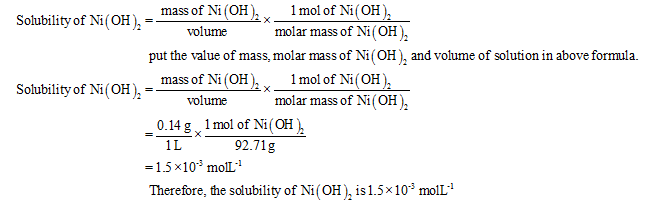# approximately  0.14 g nickel(II) hydroxide, Ni(OH)2(s), dissolved  per liter of water at 20 degree Celsius.  Calculate  Ksp for Ni(OH)2(s) at this temperature.

Question
114 views

approximately  0.14 g nickel(II) hydroxide, Ni(OH)2(s), dissolved  per liter of water at 20 degree Celsius.  Calculate  Ksp for Ni(OH)2(s) at this temperature.

check_circle

Step 1

The solubility product constant (Ksp) represents the solubility of products at equilibrium for solids in an aqueous solution.

Step 2

Given information:

Mass of Ni(OH)2 = 0.14 g molL-1

Volume of solution = 1L

Molar mass of Ni(OH)2 = 92.71 g

Step 3

Formula for calculating the solubility is shown below:...

### Want to see the full answer?

See Solution

#### Want to see this answer and more?

Solutions are written by subject experts who are available 24/7. Questions are typically answered within 1 hour.*

See Solution
*Response times may vary by subject and question.
Tagged in